MathScore EduFighter is one of the best math games on the Internet today. You can start playing for free!

## Line Segments - Sample Math Practice Problems

The math problems below can be generated by MathScore.com, a math practice program for schools and individual families. References to complexity and mode refer to the overall difficulty of the problems as they appear in the main program. In the main program, all problems are automatically graded and the difficulty adapts dynamically based on performance. Answers to these sample questions appear at the bottom of the page. This page does not grade your responses.

See some of our other supported math practice problems.

### Complexity=1, Mode=straight

Find the length of the line segment.

 1.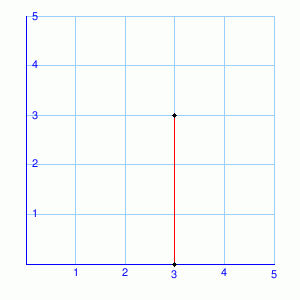Length: 2.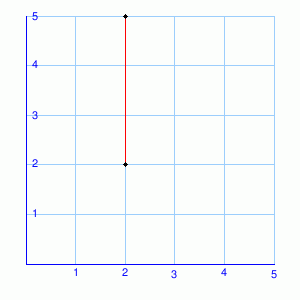Length:

### Complexity=2, Mode=straight

Find the length of the line segment.

 1.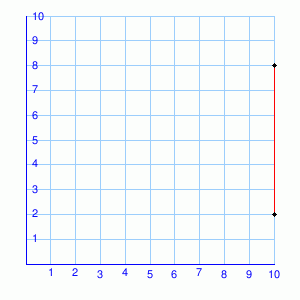Length: 2.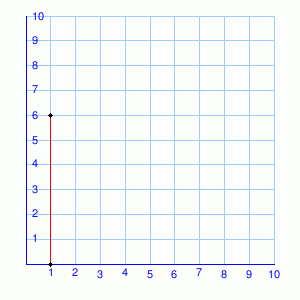Length:

### Complexity=1, Mode=straight-neg

Find the length of the line segment.

 1.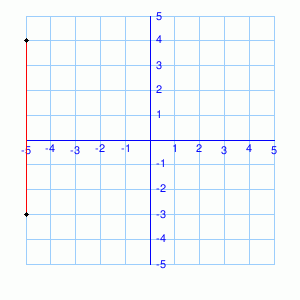Length: 2.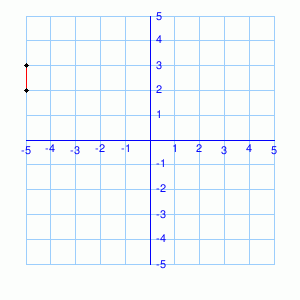Length:

### Complexity=2, Mode=straight-neg

Find the length of the line segment.

 1.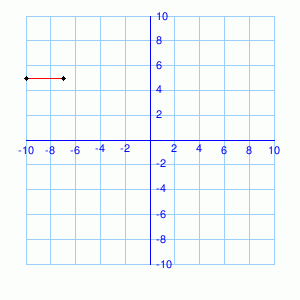Length: 2.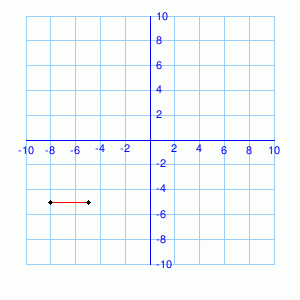Length:

### Complexity=3, Mode=straight-neg

Find the length of the line segment.

 1.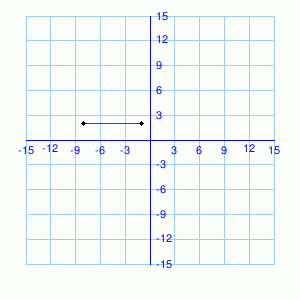Length: 2.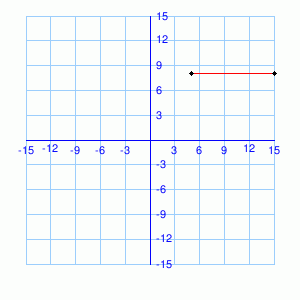Length:

### Complexity=4, Mode=straight-neg

Find the length of the line segment.

 1.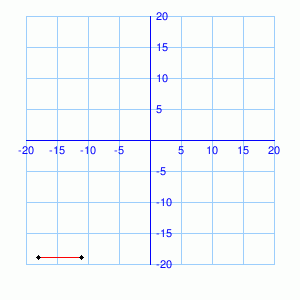Length: 2.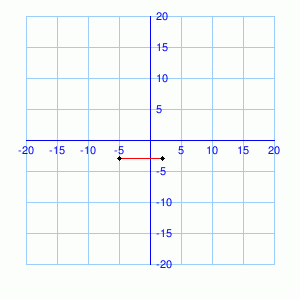Length:

### Complexity=1, Mode=straight

Find the length of the line segment.

1Length:
2Length:

### Complexity=2, Mode=straight

Find the length of the line segment.

1Length:
2Length:

### Complexity=1, Mode=straight-neg

Find the length of the line segment.

1Length:
2Length:

### Complexity=2, Mode=straight-neg

Find the length of the line segment.

1Length:
2Length:

### Complexity=3, Mode=straight-neg

Find the length of the line segment.

1Length:
2Length:

### Complexity=4, Mode=straight-neg

Find the length of the line segment.

12